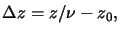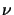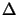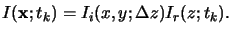Next: 5.2.3 Transfer Matrix Method Up: 5.2 Field Calculation over Previous: 5.2.1 Vertical Propagation Method

## 5.2.2 Scaled Defocus Method

The first attempt to correct the deficiencies of the vertical propagation method was made by Douglas Bernard  and, independently, by Chris Mack . They took the continuous change of the aerial image while propagating through the resist into account. For example, even in air, the aerial image depends on the vertical position with respect to the Gaussian focal plane (cf. Section 4.2.1). Denoting the focus offset in air by z0 and z as the position within the resist, the refractive effect of the air/resist interface can then be described to first-order by the net defocus(5.25)

wherebyis the real part of the refractive index n(x;tk) of the resist (cf. (5.20)).b The aerial image is now calculated for the net defocusz and then multiplied with the standing wave intensity Ir(z;tk) to obtain the bulk intensity I(x;tk), i.e.,(5.26)

For the calculation of Ir(z;tk) the same methods as for the vertical propagation model can be applied. The scaled defocus method is nowadays the default exposure model of many lithography simulators, e.g., PROLITH , SOLID , and SPESA . However, because of the separation approach the dependence of the reflection and refraction on the angle of incidence is again neglected. Thus the scaled defocus method is also restricted to low numerical apertures.

#### Footnotes

...)).b
Note that in air the refractive index has a real part of unity, i.e.,= 1.Next: 5.2.3 Transfer Matrix Method Up: 5.2 Field Calculation over Previous: 5.2.1 Vertical Propagation Method
Heinrich Kirchauer, Institute for Microelectronics, TU Vienna
1998-04-17